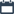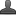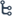## Sunday, October 25, 2020

•October 25, 2020
••, , ,
•1 comment

## Important questions for 10th class Maths chapter-3 polynomial,Mathematics questionnaire-x class

3. POLYNOMIALS

Hi friends and my dear students! In this post, I have covered Important questions for 10th class maths chapter-3 polynomial,Chapter wise previous year Questions. After Reading   Please do share it with your friends. Learn maths  for All classes here

PRIORITY-I

1 MARK QUESTIONS:

1. Give an example for

i) linear polynomial

iii) Cubic polynomial

2. Write the general form of a first degree polynomial in one variable x?

3. Define zeroes of polynomial?

4. If p(x) = 5x7 – 6x5 + 7x – 6 then find

i) coefficient of x5?

ii) degree of p(x)?

iii. constant term.

5. Write the polynomial that has

i)1 zero ?

ii) 2 zeroes?

iii) no zero

6. How will you verify if polynomial has only one zero?

7. Find the number of zeroes of

(i) 2x+1 (ii) x2 – 1 (iii) x3

8. Find the sum of the zeroes and product of zeroes of ax2 + bx + c?

2 MARK QUESTIONS:

9. If p(x) = x2 – 5x – 6 the value of find p(0), p(1), p(2), p(3),p(-1),p(-2),p(-3)?

10. If p(m) = m2 – 3m + 1 find the value of p(1) and p(-1)?

11. Check whether -3 and 3 are the zeroes of the polynomial x2 – 9?

12. Check whether -2 and 3 are the zeroes of the polynomial p(x) = x2 -x– 6?

13. Find the zeroes of the polynomial i) p(x) = x2 +5x +6 ii) p(x) = (x+2)(x-3) iii) p(x) = x4 – 16

14. Why are ¼ and –1 zeroes of the polynomials p(x) = 4x2 + 3x – 1?

15. Find the zeroes of the polynomial p(x) = x2 +7x +10 and verify the relationship between the zeroes and coefficients?

16. Draw the rough sketch of y=x3

17. Find the zeroes of the polynomial p(x) = x2 - 3 and verify the relationship between the zeroes and coefficients?

18. Find a quadratic polynomial, the sum and product of whose zeroes are -3 and 2 respectively?

19. Find quadratic polynomial if the zeroes of it are 2 and -1/3 respectively?

20. Divide 2x2 + 3x + 1 by x + 2?

21. Divide 3x3 + x2 + 2x + 5 by 1+ 2x + x2?

Also Check

Introduction To Trigonometry

Introduction to Knowing Our Numbers Key Points

SSC (10th class) Trigonometry Exercise - 11.1 Solution

SSC(10th class) Trigonometry Exercise - 11.1 Solutions

4 MARK QUESTIONS:

1. Draw the graphs of the given polynomial and find the zeroes. Justify the answers.

(i) p(x) = x2x – 12 (ii) p(x) = x2 – 6x + 9

(iii) p(x) = x2 – 4x + 5 (iv) p(x) = x2 + 3x-4 (v) p(x) = x2 – 1 (vi) x2 – 3x – 4

2. Draw the graphs of (i) y = x2x – 6 (ii) y = 6 – x x2 and find zeroes in each case. What do you notice?

3. Verify that 1, –1 and –3 are the zeroes of the cubic polynomial x3 + 3x2x – 3 and check the relationship between zeroes and the coefficients

4. Find all the zeroes of 2x4 – 3x3 – 3x2 + 6x – 2, if you know that two of its zeroes are and - 2,2

PRIORITY-II

1 MARK QUESTIONS:

1. Write the division algorithm?

2. If R(x)=x2-10x+40 then find R(1)+R(0)

3. Write the condition for ax2+bx+c is not to be a quadratic polynomial

4. Write the degree of i. x3+7x2+1 ii. x-x7+3

6. What is the degree of a zero polynomial?

7. Find the product of zeros of 2017 x2-1

8. Find the sum and product of the zeroes of p(x)=6x2-7x+3

9. What is the shape of graph of quadratic polynomial and linear polynomial?

10. When the upward parabola forms a quadratic polynomial graph?

11. Write a general polynomial of degree ‘n’ with coefficients that are a0,a1----an

12. If β,α are zeroes of the polynomial 2x2+7x+5, find the value of β+α +βα

Also Check

Important questions for 10th class maths chapter-1 Real numbers

Important questions for 10th class maths chapter-4 LINEAR EQUATIONS

2 MARK QUESTIONS:

1. Find the value of ‘k’ so that x3-3x2+4x+k is exactly divisible by x-2

2. If are the zeros of p(x)=x3+x2+ 2/3x+1 then find the βα + βδ +αδ

3. If β and α are the zeros of 2x2+x-1 then find the value of 1/α+1/ β

4. If p(x)=3x2+5x-6 then find p(1) and p(2)

5. Find a quadratic polynomial with the sum ¼ and product -1 of its zeros

6. Find the value of ‘m’ in order that x4- 2x3+3x2-mx+6 may be divisible by x-3

7. If β,α,δ are the zeroes of a polynomial of degree 3, then give the relations between the zeroes and the coeffients of the polynomial

4 MARKS QUESTIONS:

1. Draw the graph of y = x3 - 4x, find the zero of y = x3 - 4x?

2. Verify that 3, –1, -1/3 are the zeroes of the cubic polynomial p(x) = 3x3 – 5x2 – 11x – 3, and then verify the relationship between the zeroes and the coefficients

3. On dividing x3 – 3x2 + x + 2 by a polynomial g(x), the quotient and remainder were x – 2 and – 2x + 4, respectively. Find g(x)

4. Divide 3x2 – x3 – 3x + 5 by x – 1 – x2, and verify the division algorithm?

5. Obtain all other zeroes of 3x4 + 6x3 – 2x2 – 10x – 5, if two of its zeroes are and  – √5/3 ,√5/3

#### 1 comment:

1.It is what I was searching for is really informative. It is a significant and useful article for us. Thankful to you for sharing an article like this.after school program